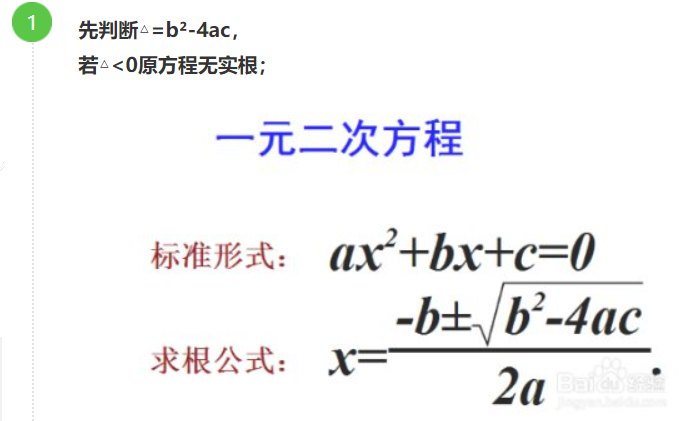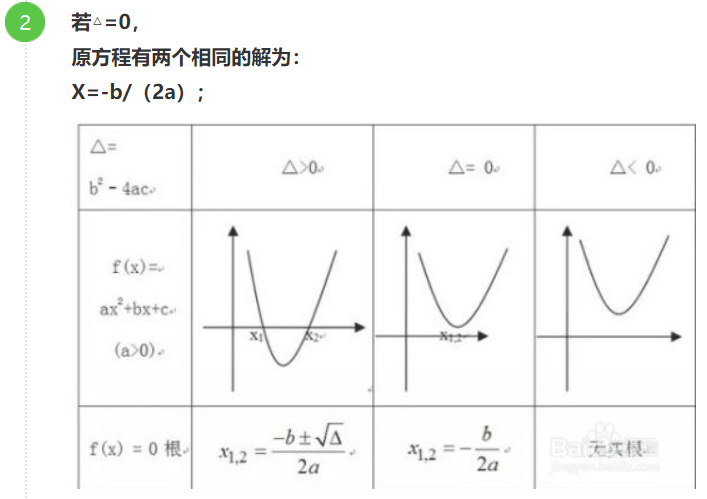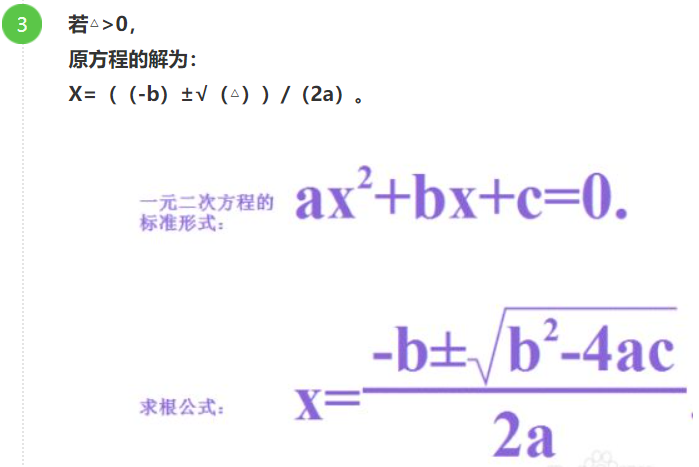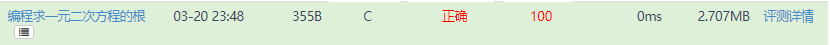• 编程求解一元二次方程
千次阅读
2017-10-06 18:23:43
#define EXP 0.00000001
int main()
{
float a = 0.0, b = 0.0, c = 0.0;
scanf("%f%f%f", &a, &b, &c);
float disc = b*b - 4 * a*c;
if ((a>-EXP) && (a<EXP))
{
printf("不是一元二次方程！\n");
}
else
{
if (disc > -EXP && disc < EXP)
{
printf("有相同解%f\n", -b / (2 * a));
}
else if (disc>0.0)
{
printf("方程有俩解%f，%f\n", (-b + sqrt(disc)) / (2 * a), (-b - sqrt(disc)) / (2 * a));
}
else
{
printf("方程无解！\n");
}
}
return 0;

}C语言
更多相关内容
• 本文实例讲述了Python编程实现数学运算求一元二次方程的实根算法。分享给大家供大家参考，具体如下： 问题： 请定义一个函数quadratic(a, b, c)，接收3个参数，返回一元二次方程：ax² + bx + c = 0的两个解。 实现...
• 文章目录一、一元二方程求解公式二、编程求解一元二次方程1、直接求解一元二次方程2、编写一元二次方程求解函数3、编写一元二次方程类 一、一元二方程求解公式 对于一元二次方程ax2+bx+c=0ax^2+bx+c=0ax2+bx+c=0 ...

# 一、一元二方程求解公式

对于一元二次方程 a x 2 + b x + c = 0 ax^2+bx+c=0

展开全文• python编程求解一元二次方程问题分析.doc
• 编程求解一元二次方程：请你编写一个程序，使其能出多个二次方程的根。该程序要询问用户是否想继续解下一个方程。用户输入1来继续，输入其它数字，则终止程序。程序要求用户输入a，b和c 一元二次方程的定义为：ax...

编程求解一元二次方程：请你编写一个程序，使其能求出多个二次方程的根。该程序要询问用户是否想继续解下一个方程。用户输入1来继续，输入其它数字，则终止程序。程序要求用户输入a，b和c

一元二次方程的定义为：ax^2+bx+c=0

显然最开始要对它进行一个判断是否存在实根，写一个函数，用一个if便可轻易实现：if ((b*b - 4 * a*c) >= 0)

如果成立输出两个实根即可，根号的符号为sqrt（）>>两个实根分别为：(((-b) + (sqrt(b*b - 4 * a*c))) / (2 * a))，(((-b) - (sqrt(b*b - 4 * a*c))) / (2 * a))

如果不成立直接cout<<"错误"<<endl;就好

接下来，要求用户多次输入数据（如果为1的话）及需要写一个递归，在函数里再加上一个if进行判断用户最后输入的数是否为1即可，如果为1：就让用户接着输入数据，并调用这个函数自身进行实根判断，输出，如此循环便可达到题目要求。

直接上代码：

#include "pch.h"
#include <iostream>
using namespace std;
void funA(double a, double b, double c, int d)
{
if ((b*b - 4 * a*c) >= 0)
cout<<"结果为：" << (((-b) + (sqrt(b*b - 4 * a*c))) / (2 * a)) << "  " << (((-b) - (sqrt(b*b - 4 * a*c))) / (2 * a)) << endl;
else
cout << "错误" << endl;
if (d == 1)
{
cout << "please cin four number:" << endl,
cin >> a >> b >> c >> d,
cout<<"结果为：",
funA(a, b, c, d),
cout << endl;
}
else cout << "surprise motherfuck!" << endl;

}
int main()
{
double a, b, c;
int d;
cout << "please cin four number as‘double, double, double, int’:" << endl;
cin >> a >> b >> c >> d;
funA(a, b, c, d);
cout << endl;
return 0;
}

展开全文函数
• 　编写一个求解一元二次方程的实数根的程序，方程的系数由用户在运行xh 输入格式 　输入一行三个整数分别为一元二次方程的三个系数，数据之间以空格隔开 输出格式 　输出一行为方程的实数根（若两根不同 较大的在前 ...
问题描述
编写一个求解一元二次方程的实数根的程序，方程的系数由用户在运行xh
输入格式
输入一行三个整数分别为一元二次方程的三个系数，数据之间以空格隔开
输出格式
输出一行为方程的实数根（若两根不同 较大的在前 两根以空格隔开 若两根相同 输出一个 若无根 输出 NO ）
样例输入
1 -5 4
样例输出
4 1
样例输入
1 -2 1
样例输出
1
样例输入
1 0 1
样例输出
NO


题解思路：

公式法：代码如下：

#include<stdio.h>
#include<math.h>
int main() {
double a,b,c;
scanf("%lf%lf%lf",&a,&b,&c);
double root=0,x1=0,x2=0;
root=b*b-4*a*c;
if(root>0){
x1=(-b+sqrt(root))/(2*a);
x2=(-b-sqrt(root))/(2*a);
if(x1>x2){
printf("%g %g",x1,x2);
}
}else if(root==0){
x1=(-b)/(2*a);
x2=x1;
printf("%g",x1);
}else{
printf("NO");
}

return 0;
}本题要注意一个精度输出，不然难过一些事数据。比如2 5 1.

printf("%g %g",x1,x2);


此行代码中%g是什么意思。
%g是C语言printf()函数的一个输出格式类型，它表示以%f%e中较短的输出宽度输出单、双精度实数，在指数小于-4或者大于等于精度时使用%e格式。

举个例子

printf("%g\n", 0.00001234);

printf("%g\n", 0.0001234);

printf("%.2g\n", 123.45);

printf("%.2g\n", 23.45);


结果：

1.234e-05
0.0001234
1.2e+02
23

展开全文• #include #include void main() { ... printf("请输入一元二次方程的系数a，b，c的值：\n"); scanf("%f,%f,%f",&a,&b,&c); if(a==0 && b==0) printf("该方程无解!\n"); else if(a==0 && b!=0)
• 要求：从键盘上输入一元二次方程的三个参数，编程判断并求一元二次方程的实根(a,b,c均为整数)算法分析：一元二次方程是只含有一个未知数，且未知数的最高次数是二次的多项式方程。一元二次方程经过整理都可化成一般...
• 编写一个求解一元二次方程的实数根的程序，方程的系数由用户在运行xh 输入格式 输入一行三个整数分别为一元二次方程的三个系数，数据之间以空格隔开 输出格式 输出一行为方程的实数根（若两根不同 较大的在前 两根以...
• 首先如图所示敲出下列代码： 即如下 import math a = float(input("请输入a的值：")) b = float(input("请输入b的值：")) c = float(input("请输入c的值："))if a !... delta = b**2-4*a*c ... s ...python 开发语言 后端
• Labview编写的进行一元二次方程的计算，可设置不同的系数进行计算。labview
• 该楼层疑似违规已被系统折叠 隐藏此楼查看此楼 头文件#if! defined(EQUATION_H)#define EQUATION_H#include #include using namespace std; class FindRoot{private:float a,b,c,d...cout一个一元二次方程根的程序"
•python 开发语言 后端
• 对于简单的一元次方程的迭代#include #include #include #define MAXTIMES 5typedef int times;typedef double coefficient;typedef struct _properties{coefficient x; //系数times n;//次数}...
• 界面的abc输入托文本框获取把Private Sub Command1_Click() 。Dim a As Single, b As Single, c ...含义及特点：(1)一元二次方程的解(根)的意义：能使一元二次方程左右两边相等的未知数的值称为一元二次方程的解。一...
• 前情提要：在求解一元二次方程的时候可以使用根公式。 即：如aX^2 + bX + c = 0则 代码如下：[以下代码中的\n \t为个人喜好，可自行更改] #define _CRT_SECURE_NO_WARNINGS #include<stdio.h> #...c++ c#
• python：求解一元二次方程 工具：spyder3 环境：Windows10、python3.6 目的：对基本数据类型、输入输出进行基础复习 #coding=utf8 import math a=int(input("请输入a：")); b=int(input("请输入b：")); c=int(input...python
• 试题 算法提高 编程求一元二次方程的根 资源限制 时间限制：1.0s 内存限制：256.0MB 问题描述 　编写一个求解一元二次方程的实数根的程序，方程的系数由用户在运行xh 输入格式 　输入一行三个整数分别为一元二次方程...c++
• using System; using System.Collections.Generic; using System.Linq; using System.Text; namespace ConsoleApplication1 ... static void Main(string[] args) ...总结：我通过这次学习懂得了编程求一元二次方程
• 1.fabs();是绝对值函数，例如：fabs(n)<1e-6,其中e表示10，1e-6表示10的(-6)...//求一元二次方程的根 #include <iostream> #include <math.h> using namespace std; int main() { float a, b, c,d...大数据 c++
• 配方法(直接开)形如x=p或(nx+m)=p(p≥0)的一元二次方程可采用直接开平方的方法解一元二次方程．如果方程化成x²=p的形式，那么可得x=±p；(x²=p,x=±根号p)如果方程能化成(nx+m)=p(p≥0)的形式，那么nx+m=±p．...
•c++
•c语言 算法 c++
• 下面我们就来编写定义一个简单的函数来求解一元二次方程吧。 1 问题 定义一个函数，quadratic（a，b，c），接收三个参数，返回一元二次方程ax2+bx+c=0的两个解。 2 方法 使用def语句编写函数，依次写出函数名，括号...python 算法 人工智能 深度学习
• 这里用到了数学里解一元二次方程根公式 // 一元二次方程 ... Console.WriteLine("求解一元二次方程ax*x+bx+c=0"); Console.WriteLine("请输入a："); a = Convert.ToDouble(Console.ReadLine()c# linq 开发语言...